# Civil Engineering - Applied Mechanics

### Exercise :: Applied Mechanics - Section 5

41.

The moment of inertia of a triangular section (base b, height h) about centroidal axis parallel to the base, is

 A.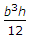B.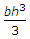C.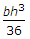D.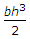E.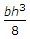Explanation:

No answer description available for this question. Let us discuss.

42.

The horizontal reaction at the support A of the structure shown in below figure, is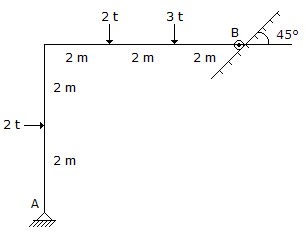A. zero B. 1 t C. 2 t D. 3 t E. 2.5 t.

Explanation:

No answer description available for this question. Let us discuss.

43.

Periodic time of body moving with simple harmonic motion, is

 A. directly proportional to its angular velocity B. directly porportional to the square of its angular velocity C. inversly proportional to the square of its angular velocity D. inversely proportional to its angular velocity.

Explanation:

No answer description available for this question. Let us discuss.

44.

On a ladder resting on a rough ground and leaning against a smooth vertical wall, the force of friction acts

 A. downwards at its upper end B. upwards at its upper end C. perpendicular to the wall at its upper end D. zero at its upper end E. none of these.

Explanation:

No answer description available for this question. Let us discuss.

45.

The centre of gravity of a plane lamina will not be at its geometrical centre if it is a

 A. circle B. equilateral triangle C. rectangle D. square E. right angled triangle.